## ICSE Physics Previous Year Question Paper 2015 Solved for Class 10

ICSE Paper 2015
PHYSICS

(Two hours)
Answers to this Paper must he written on the paper provided separately.
You will not be allowed to write during the first 15 minutes.
This time is to he spent in reading the question paper.
The time given at the head of this Paper is the time allowed for writing the answers.
Section I is compulsory. Attempt any four questions from Section II.
The intended marks for questions or parts of questions are given in brackets [ ].

Question 1:
(a) When a body is placed on a table top, it exerts a force equal to its weight downwards on the table top but does not move or fall.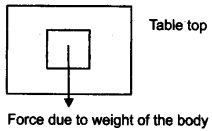(i) Name the force exerted by the table top.
(ii) What is the direction of the force ?  
(b) (i) Name one factor that affects the lateral displacement of light as it passes through a rectangular glass slab.
(ii) On reversing the direction of the current in a wire, the magnetic field produced by it gets ………. .  
(c) (i) On what factor does the position of the centre of gravity of a body depend ?
(ii) What is the S.I. unit of the moment of force ?  
(d) Name the factors affecting the turning effect of a body.  
(e) (i) Define equilibrium.
(ii) In a beam balance when the beam is balanced in a horizontal position, it is in ……….. equilibrium. 

(a) (i) Normal reaction force.
(ii) It acts normal to the Surface of table top i.e., opposite to the direction of the force due to the weight of the body.

(b) (i) The factors that affect the lateral displacement of a light as it passes through a rectangular glass slab are :
(1) The thickness of the glass slab: The lateral displacement increases with the increase in the thickness of the glass slab.
(2) The angle of incidence: It increases with the increase in the angle of incidence.
(3) The refractive index of glass: The wavelength of light used. (It is more for violet light than for the red light.) (any one)
(ii) ‘Reversed’.

(c) (i) The position of centre of gravity of a body of given mass depends on its shape i.e., on the distribution of mass in it.
(ii) S.I. unit of the moment of force is Newton × meter.

(d) The factors affecting turning effect of a body:
1. The magnitude of a force applied.
2. The distance of line of action of the force from the axis of rotation.

(e) (i) When a number of forces acting on a body produce no change in its state of rest or of the motion, the body is said to be in equilibrium.
(ii) Static equilibrium.

Question 2:
(a) How is work done by a force measured when the force :
(i) is in the direction of displacement.
(ii) is at an angle to the direction of displacement.  
(b) State the energy changes in the following while in use :
(i) Burning of a candle.
(ii) A steam engine.  
(c) (i) A scissor is a ………… multiplier.
(ii) 1 kWh = ……….. J.  
(d) Explain the motion of a planet around the sun in a circular path.  
(e) Rajan exerts a force of 150 N in pulling a cart at a constant speed of 10 m/s. Calculate the power exerted.  

(a) (i) Work done by a force when the force is in the direction of displacement = Force × Displacement of the point of application of the force in the direction of force.
(ii) Work done by a force when the force is at an angle [θ] to the direction of displacement = Force × component of displacement in the direction of force = Force × d cos θ
[d is the displacement of the body at the angle θ to the direction of force]

(b) Energy changes :
(i) Burning of a candle: Chemical energy to light energy.
(ii) Steam engine: Chemical energy of coal first changes to heat energy of steam and then heat energy charges into mechanical energy.

(c) (i) A scissor is a speed multiplier.
(ii) 1 kWh = 3.6 × 106 J.

(d) A planet moves around the sun in a circular path for which the gravitational force of attraction on the planet by the sun provides the necessary centripetal force. This centripetal force is always directed towards the centre of the sun at each point of its path which is responsible for circular motion of planet.

(e) Given : Force = 150 N, Speed = 10 m/s.
Power = Force × Speed
= 150 × 10
= 1500 Watt.

Question 3:
(a) (i) Give the expression for mechanical advantage of an inclined plane in terms of the length of an inclined plane.
(ii) Name a common device where a gear train is used.  
(b) The speed of light in glass is 2 × 106 km/s. What is the refractive index of glass ?  
(c) (i) Draw a graph between displacement and the time for a body executing free vibrations.
(ii) Where can a body execute free vibrations ?  
(d) (i) What happens to the resistivity of semi-conductors with the increase of temperature ?
(ii) For a fuse, higher the current rating is the fuse wire.  
(e) (i) Name the high energetic invisible electromagnetic waves which help in the study of the structure of crystals.
(ii) State an additional use of the waves mentioned in part (e) (i).  

(a) (i)(ii) Watch is the common device where a gear train is used.

(b)(c) (i)(ii) In vaccum.

(d) (i) The resistivity of semiconductor decreases with the increase in temperature.
(ii) Thicker.

(e) (i) X-rays.
(ii) X-rays are used for detection of fracture in bones, teeth etc.

Question 4:
(a) Rishi is surprised when he sees water boiling at 115°C in a container. Give reasons as to why water can boil at the above temperature. 
(b) (i) Why does a current carrying, freely suspended solenoid rest along a particular direction ?
(ii) State the direction in which it rests. 
(c) Find the equivalent resistance between points A and B.(d)
Give two similarities between an A.C. generator and a D.C. motor. 
(e)
(i) Why is a cathode ray tube evacuated to a low pressure ?
(ii) What happens if the negative potential is changed on a grid ?  

(a) Water might have some impurities (eg., salt) dissolved in it. Because the boiling point of liquid (water) increases by the addition of impurities (eg., salt) to it.

(b) (i) Current carrying freely suspended solenoid behaves just like a bar magnet with fixed polarities (North and South) at its ends. Hence it rest in North- South direction exactly in the same manner as a bar magnet does.
(ii) North-South direction.

(c)(d) (i) Both have a strong horse shoe permanent magnet or an electromagnet.
(ii) Both have an armature coil having soft iron core.

(e) (i) The cathode ray tube is evacuated to a low pressure so that the electrons may move without being obstructed by air molecules.
(ii) The variation of the negative potential on the grid affects the number of electrons reaching the anode and striking the screen changed which changes the brightness of pattern on the screen.

SECTION-II (40 Marks)

Attempt any four questions from this Section

Question 5:
(a) Draw a simplified diagram of a lemon crusher, indicating direction of load and effort. 
(b) (i) Name the physical quantity measured in terms of horse power.
(ii) A nut is opened by a wrench of length 20cm. If the least force required is 2N, find the moment of force needed to loosen the nut.
(iii) Explain briefly why the work done by a fielder when he takes a catch in a cricket match is negative. 
(c) A block and tackle system has V.R = 5.
(i) Draw a neat labelled diagram of a system indicating the direction of its load and effort.
(ii) Rohan exerts a pull of 150 Kgf. What is the maximum load he can raise with this pulley system if its efficiency = 75% ?  

(a)(b) (i) Horse power is another unit of Power.
1 H.P = 746 watt.
(ii) Given : Force = 2N, d = 20 cm = 0.2 m.
Moment of force = Force × distance
= 2 × 0.2
= 0.4 Nm.
(iii) Work done is said to be negative when a force opposes the motion or tries to stop a moving body since the fielder also tries to stop the moving ball hence his work done is also negative.

(c) (i)(ii)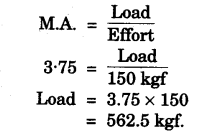Question 6:
(a) (i) Where should an object be placed so that a real and inverted image of the same size as the object is obtained using a convex lens ?
(ii) Draw a ray diagram to show the formation of the image as specified in the part a (i).  
(b) (i) Why does the Sun appear red at sunrise ?
(ii) Name the subjective property of light related to its wavelength. 
(c) Jatin puts a pencil into a glass container having water and is surprised to see the pencil in a different state.
(i) What change is observed in the appearance of the pencil ?
(ii) Name the phenomenon responsible for the change.
(iii) Draw a ray diagram showing how the eye sees the pencil. 

(a) (i) When object is placed at 2F1 (center of curvature) distance from the optical center on the principal axis of a convex lens a real and inverted image of the same size of the object is formed.
(ii)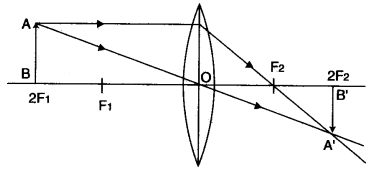(b) (i) During the sunrise the light from the sun has to travel the longest distance of atmosphere to reach the observer. The light travelling from sun loses blue light of short wavelength due to scattering, while the red light of long wave length is scattered a little. So it is not lost much. Thus blue light is almost absent in sunlight reaching the observer and red light only reaches us. So the sun is seen red.
(ii) Colour is the subjective property of light related to its wavelength.

(c) (i) The pencil under water appears to be shortened and raised up.
(ii) Refraction of light.
(iii)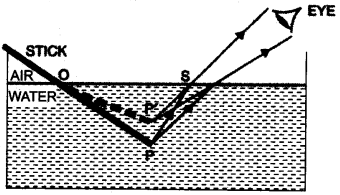Question 7:
(a) (i) State the safe limit of sound level in terms of decibel for human hearing.
(ii) Name the characteristic of sound in relation to its waveform. 
(b) A person standing between two vertical cliffs and 480 m from the nearest cliff shouts. He hears the first echo after 3s and the second echo 2s later.
Calculate:
(i) The speed of sound.
(ii) The distance of the other cliff from the person. 
(c) In the diagram below, A, B, C, D are four pendulums suspended from the same elastic string PQ. The length of A and C are equal to each other while the length of pendulum B is smaller than that of D. Pendulum A is set into a mode of vibrations.(i) Name the type of vibrations taking place in pendulums B and D ?
(ii) What is the state of pendulum C ?
(iii) State the reason for the type of vibrations in pendulums B and C. 

(a) (i) The sound level 30 dB to 10 dB has the soothing sensation.
(ii) Quality (or timbre)
(b) (i)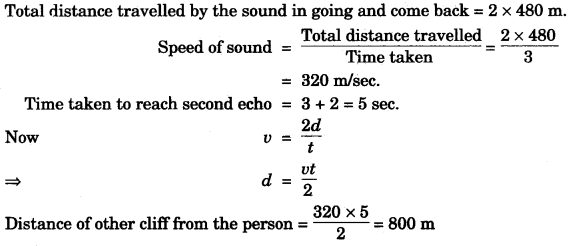(c) (i) B and D would be in the state of force vibration.
(ii) Since the length of pendulum C is same as that of A therefore it is in the state of resonance.
(iii) The pendulum C has its length same as that of A therefore its natural time period is also same that of A and both remain in same phase so resonance takes place. But B’s length is lesser than the length of A hence B would vibrate with smaller amplitude.

Question 8:
(a) (i) Name the device used to increase the voltage at a generating station.
(ii) At what frequency is A.C. supplied to residential houses ?
(iii) Name the wire in a household electrical circuit to which the switch is connected. 
(b) The relationship between the potential difference and the current in a conductor is stated in the form of a law.
(i) Name the law.
(ii) What does the slope of V-I graph for a conductor represent ?
(iii) Name the material used for making the connecting wire. 
(c) A cell of Emf 2V and internal resistance 1.2Ω is connected with an ammeter of resistance 0.8Ω and two resistors of 4.5Ω and 9Ω as shown in the diagram below :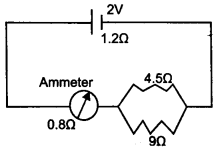(i) What would be the reading on the Ammeter ?
(ii) What is the potential difference across the terminals of the cell ? 

(a) (i) Transformer [step up transformer].
(ii) 50 Hz
(iii) Live wire.
(b) (i) Ohm’s law.
(ii) The resistance of the conductor
(iii) Copper (since it has less resistance).

(c)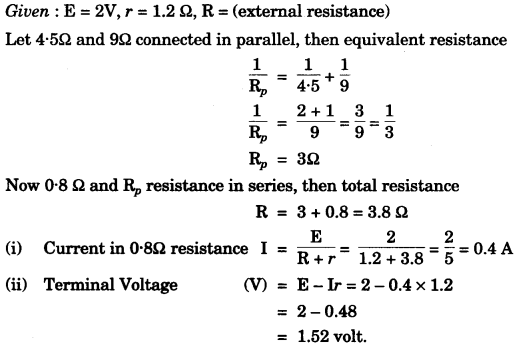Question 9:
(a) (i) Name a gas caused by the Greenhouse effect.
(ii) Which property of water makes it an effective coolant ? 
(b) (i) Water in lakes and ponds do not freeze at once in cold countries. Give a reason in support of your answer.
(ii) What is the principle of Calorimetry ?
(iii) Name the law on which this principle is based.
(iv) State the effect of an increase of impurities on the melting point of ice. 
(c) A refrigerator converts 100g of water at 20°C to ice at -10°C in 35 minutes. Calculate the average rate of heat extraction in terms of watts.
Given : Specific heat capacity of ice = 2.1 Jg1 °C1.
Specific heat capacity of water = 4.2 Jg1 °C1.
Specific Latent heat of fusion of ice = 336 Jg1. 

(a) (i) Carbon dioxide.
(ii) High specific heat capacity.

(b) (i) The specific latent heat of fusion of ice is sufficiently high (336 Jg1) so to freeze water, a large quantity of heat has to be withdrawn, hence it freezes slowly.
(ii) Heat energy lost by the hot body is equal to the heat energy gained by the cold body. This is called principle of calorimetry. For this, hot and cold body should be kept in close contact and there is no exchange of heat from surroundings.
(iii) Law of conservation of energy.
(iv) The melting point of ice decreases by increase of impurities in it.

(c)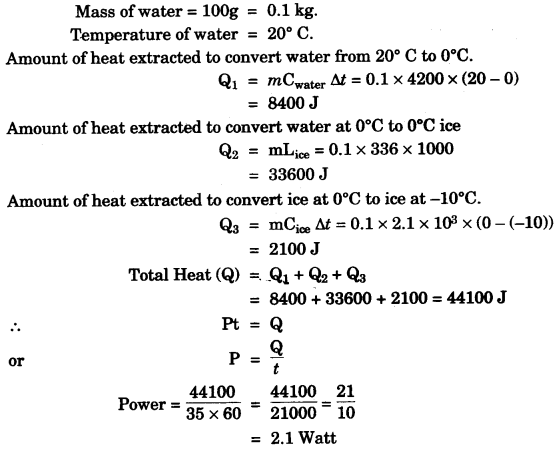Question 10:
(a) (i) What is thermionic emission ?
(ii) Name the unit in which the work function of a metal is expressed. 
(b) (i) Complete the diagram as given alongside by drawing the deflection of radioactive radiations in an electric field.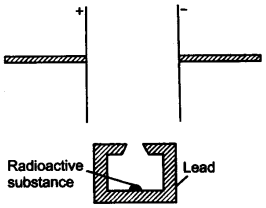(ii) State any two precautions to be taken while handling radioactive substances. 
(c) An atomic nucleus A is composed of 84 protons and 128 neutrons.
(i) The nucleus A emits an alpha particle and is transformed into nucleus B. What is the composition of nucleus B ?
(ii) The nucleus B emits a beta particle and is transformed into a nucleus C. What is the composition of nucleus C ?
(iii) Does the composition of nucleus C change if it emits gamma radiations ? 

(a) (i) The emission of electrons from a metal surface when heat (or thermal) energy is imparted to it, is called thermionic emission.
(ii) eV (electron volt); 1 eV = 1.6 x 10-11 J.

(b) (i)(b) The radio active substance are kept in a thick lead containers with a very narrow opening so as to stop radiations coming out from other direction.
(c) Radio active material should be handled with long lead tongs. (any two).

(c) (i) Given : Composition of atomic nucleus A = 84 protons and 128 neutrons.
Mass no. of A = 84 + 128 = 212 Atomic no. of A = 84
The nucleus A emits an alpha particle, the mass number decreases by 4 and the atomic no. decreases by 2.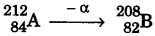(ii) No. of protons in B = 82.
No. of neutrons in B = 208 – 82 = 126.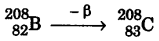No. of protons in C = 83
No. of neutrons in C = 208 – 83 = 125
(iii) NO.